视觉slam14讲——第11讲后端2

• 理解Pose Graph 优化
• 因子图优化
• 增量式图优化

1 位姿图(Pose Graph)

1.1 Pose Graph的意义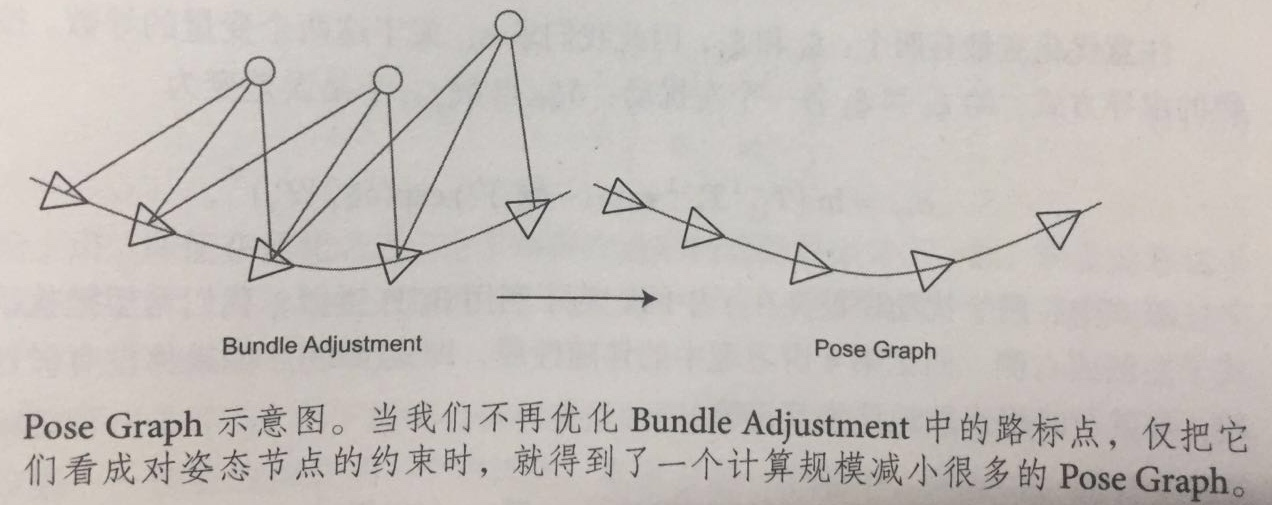1.2 Pose Graph优化推导

Pose Graph节点表示相机位姿，用ξ1,...,ξn$\xi_1,...,\xi_n$表达，边是两个位姿节点之间的相对运动估计，这种估计可能来自于特征点法或者直接法，称ξiξj$\xi_i和\xi_j$之间运动为ξij$\triangle\xi_{ij}$

ξij=ξ1iξj=ln(exp((ξi)^)exp(ξ^j))

eij=ln(exp((ξij)^)exp((ξi)^)exp(ξ^j))

minξ=12i,jεeTi,ji,j1ei,j

2 因子图优化

2.1 贝叶斯网络Bayes Network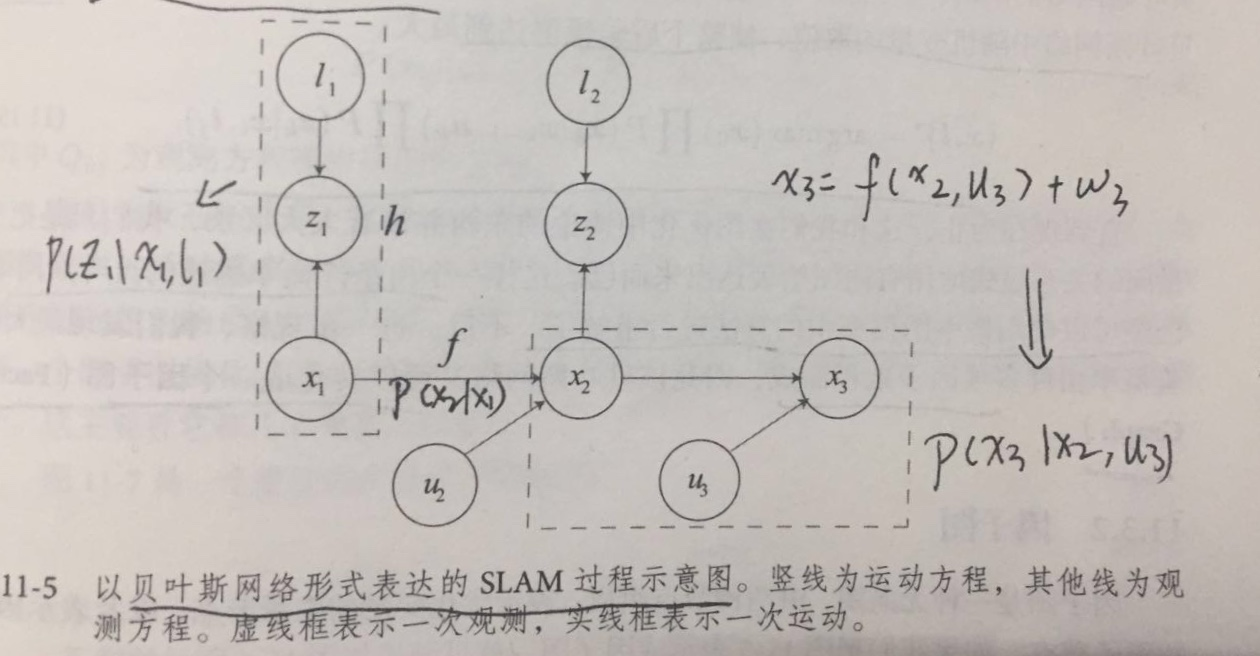{x,l}=arg max(x0)P(xk|xk1,μk)P(zk|xi,lj)

2.2 因子图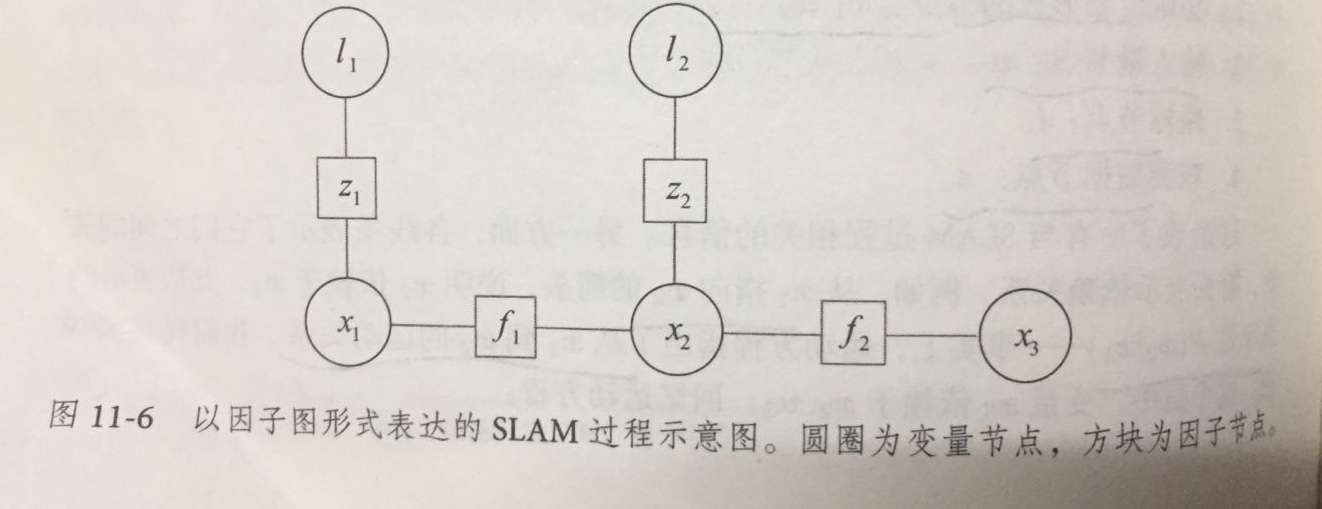2.3 增量特性

Kaess等人提出的iSAM(incremental Smooth and Mapping)中，对因子图进行了更加精细的处理，使得它可以增量式的处理后端优化。

J(x)=keTv,kR1kev,k+kjeTy,k,jQ1k,jey,k,j

Hx=g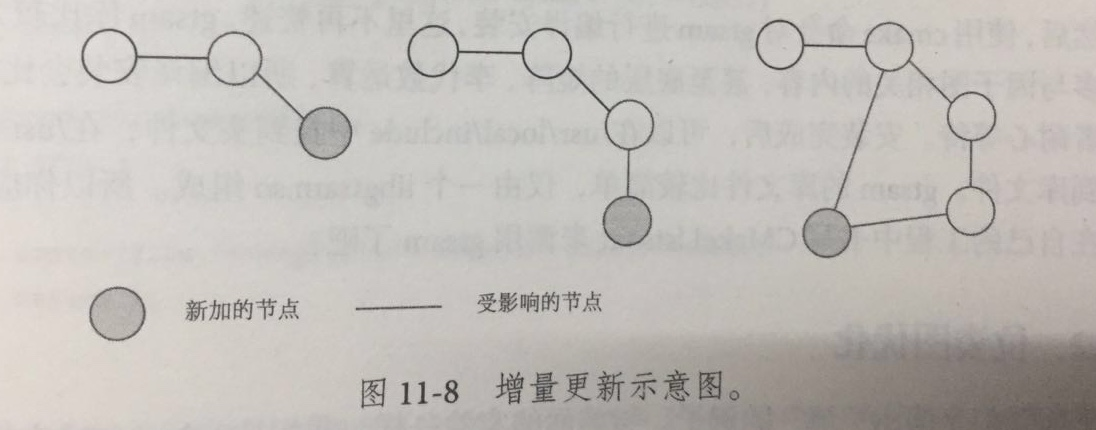3 后端优化代码实践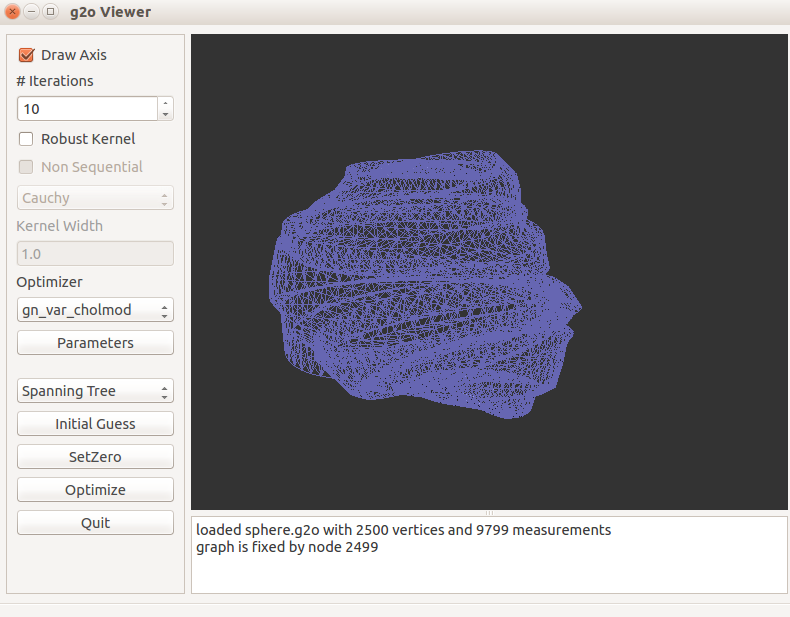3.1 使用g2o/types/slam3d/中的SE3表示位姿图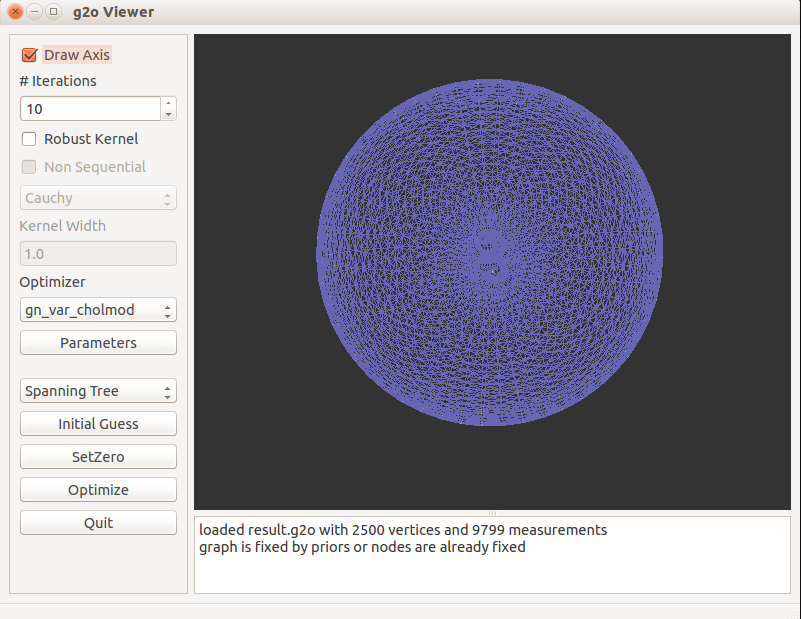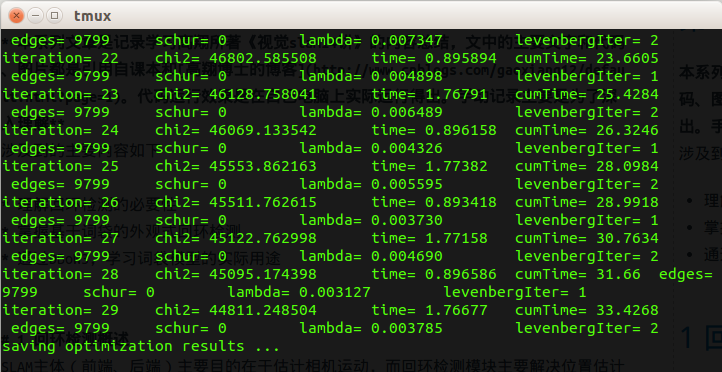3.2 使用李代数表达位姿图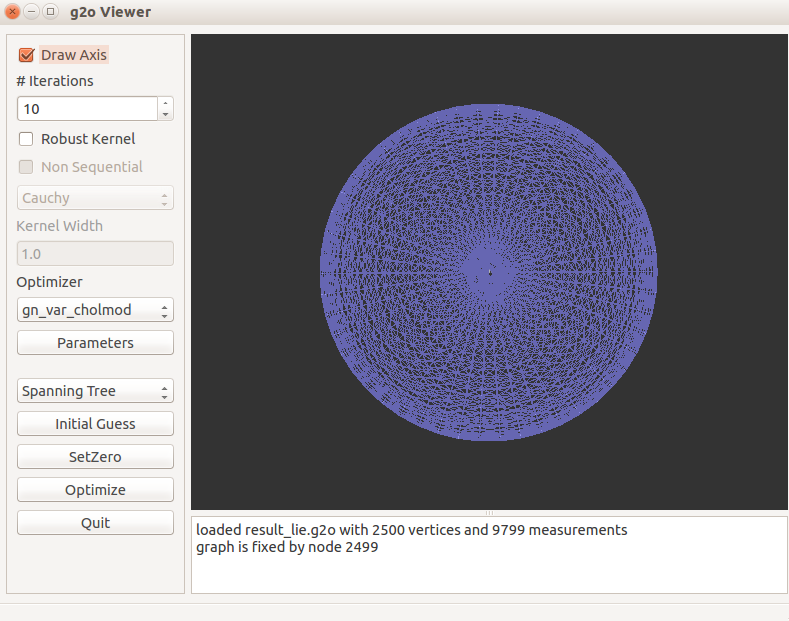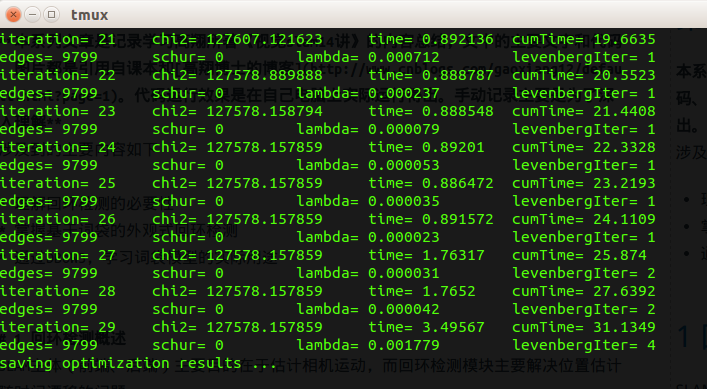3.3 使用gtsam 中的因子图表达位姿图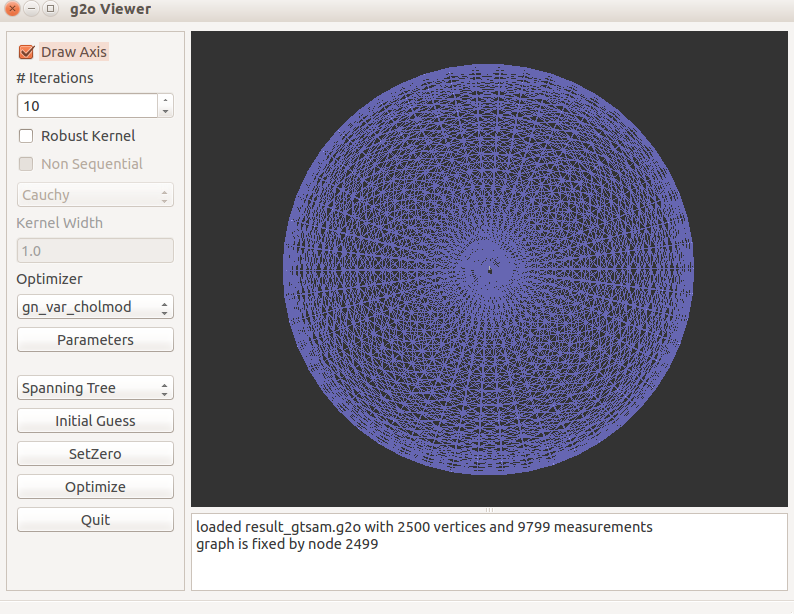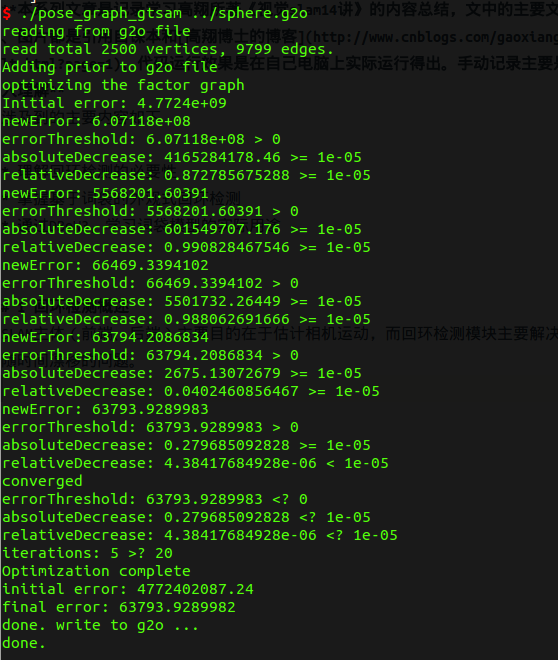3.4 总结